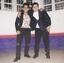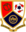Trending ▼   ResFinder# ICSE Class X Prelims 2019 : Computer Applications (Villa Theresa High School (VTS), Mumbai)

4 pages, 39 questions, 18 questions with responses, 18 total responses,20Jash Popat Campion School, Mumbai
+Fave Message
 Home > jash04 >   F Also featured on: School Page icseFormatting page ...

J T n AU i0 0 L T H s c T H E R B A H 0 \ m v u m h ry c n n Z W lb n n Z \n \c r \N kj n lm 4 r r l I 4 P r r l * T A m ) A m h \ t tn w o 4 w * n T h n w p w ' v l n /= n hr q n m A t n p t n k ru t n b pr m u \ r . . w r + l t h w w r r \ ! l , I q w Jm r qw r l l l v ll r n ht H \ ' n o n r n r r pn d tl r n r \ s t w r c n w ir d Ji r p r u tt l in w r i n g h a n B n iM n n w q n l n l n s r c la A n f n n m tm m m t w r w tm m T std m b r o c t I u O N A 44 0 M a r ) SECT u w A n r f q } in n iw ha t o n u es ce o l ite r a ls W n an is th c d iffe r c n a c c es s rr a n ce fo llo ge c 12 \ spcci? w n w in g \ o rs in th e ir e r a n d S tllr r a n 12 1 ( To c k To c r e a te a n o h a n g e th e M q u sc o d p r fu a te f o p r r o lo r s ? A f p b lic l p rec ed en fin e ( - 0 ( )W a t is th c w H ,c c ha r a c te r e w b e c m h a r o b ile ilh i in th e S tr in g B fo r c la s s e lie r e le c ln o n ic 4 p h a be f ' " 2 p O) T h s Pa pe r c o n ta in s 4 p le d pa ges T m u O ve r Scanned by CamScannerFormatting page ...

Top Contributors
to this ResPaperVehaan Handa(17)Disha Lohia(1)hanishajain2003(1)Dhrumil Parekh(1)Formatting page ...Formatting page ...